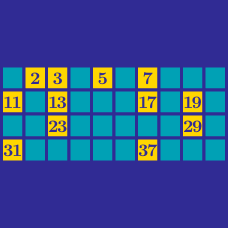Number Theory

# Prime Numbers Warmup

What is the largest even prime number?

A teacher would like to have their students work together in small groups of at least 2. If there are 23 students and each student can only be in one group, is it possible to divide the students into at least 2 groups such that all the groups have the same number of students?

8 can be written as the sum of 2 prime numbers: $8 = 3 + 5$ Which of the following numbers cannot be written as the sum of 2 prime numbers?

3, 5, and 7 are all prime. Is there an $n > 3$ such that $n,$ $n + 2,$ and $n + 4$ are all prime?

Is there a positive integer $n$ such that $6n + 15$ is prime?

×